# Calculating Ideal Spring and Roll Bar Rates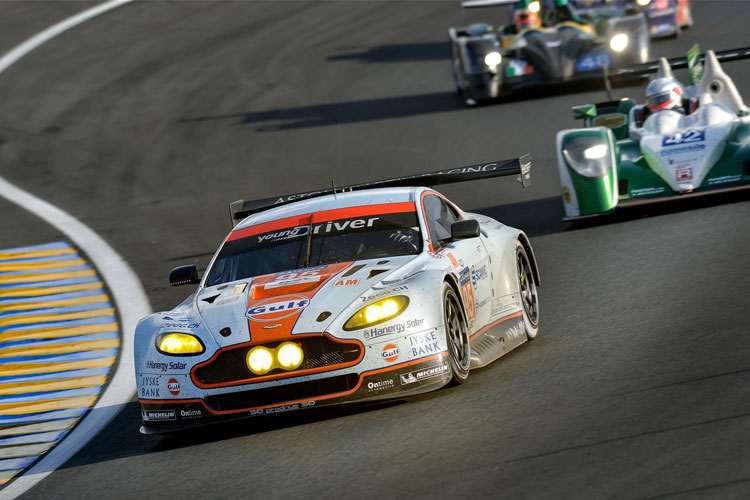The ride and roll rates of your car determine a change in wheel load to a change in body position, be that in bump or roll. Roll rates determine the change in tyre normal force side to side for a given applied torque to the chassis or a given amount of chassis roll. Ride rates determine the change in tyre normal force for one wheel into a pure vertical change in body position.

One of the main purposes of suspension is to generate as much grip as possible from the tyres in order to make the car go as fast as possible. There are other uses in other sectors of automotive such as comfort and speed bumps etc but for this section we will be focusing on track cars and motorsport vehicles that only have the need to generate maximum grip from their car.

One issue with calculating ideal spring rates for a car is that calculations are based on ideal wheel loadings of the car. However, the ideal wheel loadings require ideal spring rates to calculate from. Therefore, a typical design problem is formed. In order to overcome this we need to assume some aspects of the suspension system initially that we can then return to once we have our initial figures to fine tune.Throughout this section we will have to make some assumptions which will be explained throughout.

Stage 1: The AssumptionsInitial assumptions of this section are that the car has zero degrees of camber and that camber does not change with ride. This is required to avoid developing very complex equations in this section. We also have to assume that no tyre lateral distortion occurs. First of all ride rates need to be determined for the suspension system.

To get initial ride rates you can either begin with a desired frequency or with maximum travel divided by maximum load. Either option will work accurately. Usually it is best to have the suspension as soft as possible in light of vehicle load, cornering forces, roll stiffness and roll stiffness distribution. Race cars are known for being stiff; despite being stiff they are as soft as they can be whilst still providing good cornering characteristics, optimum wheel loadings and full suspension/ damper range to work the suspension as efficiently as possible. For aero cars the suspension is often much stiffer to avoid changes in ride height affecting the downforce of the car constantly.

The equation below can be used to find optimum un-damped frequency based upon the available wheel movement that is available in bump and droop from ride height. To check your results or to use the following assumptions for your car the below figures have been acquired from motorsport data and can be used as a rule of thumb for your category of car.

If you have an ideal vertical wheel movement in bump and droop then the below equation can be used to convert it to frequency:Where:

ω = Frequency in cycles per minute

x = Vertical wheel displacement from ride height in metres

Typical Ride Frequencies:

• Passenger Cars: 30-50 Cycles per minute
• Sports Cars: 70-90 Cycles per minute
• Open Wheel Formula Car without Ground Effect: 95-120 Cycles per minute
• Vehicles with High Ground Effect: Several hundred Cycles per minute dependant on downforce level.

Alternatively there are figures that can be used for the wheel travels expected in classes of vehicles:

• Passenger Cars: +/- 10cm
• Sports Cars and Open Wheel Cars Without High Downforce: +/- 4cm to +/-8cm
• Car with High Levels of Ground Effect: +/- 1.5cm or sometimes less.

Now we need to generate a figure to work with for our roll gradient. The roll gradient of a car is the amount of degrees of roll generated per g of lateral acceleration.  Again we can use some typical values from motorsport data shown below to get the process going.

• Large Passenger Cars: 7.0 degrees/g
• Small Passenger Cars: 5.0 Degrees/g
• Sports cars: 4.2 Degrees/g
• High Performance Road Cars and Track Day Cars: 3.0 Degrees/g
• Purpose Built Racing Cars: 1.5 Degrees/g
• Active-Servo Controlled Stiffness: 0 Degrees of roll or as close to

Now we must gather some data of the car in order to begin running calculations using the above values. The first run of equations will be on a car with independent suspension. The solution worked below is an open loop system where initial assumptions must first be made and worked through. The first set will likely be off slightly so we can then re-run with altered figures based on our first outcome to close in on the ideal ride and roll rates.

The conditions that we are going to assume for our car are all listed below. This car is left/right symmetric and is independently suspended. The aerodynamic lift, rolling moment co-efficients, drivetrain torque and longitudinal acceleration are all zero for this exact point of investigation in the middle section of the corner. The car has the below data:

Weight:

• W1 = 460Kg
• W2 = 460 Kg
• W3 = 310 Kg
• W4 = 310Kg

Therefore:

• W (front) =920Kg
• W (rear) = 620Kg
• W (total) = 1540Kg

Required Dimensions:

• Front Track Width (tf) = 1.5m
• Rear Track Width (tr) = 1.5m
• Wheel Base (L) = 2.9m
• Centre of Gravity Height (h) = 0.457m
• Centre of Gravity to Roll Axis Height (H) = 0.335m

The cornering conditions are:

• Corner Direction = Left Hand
• Cornering Path Radius (R) = -180m
• Cornering Velocity = 100mph = 44.7m/s

Roll axis heights above ground are:

• Front Roll Axis Height (Zrf) = 0.0762m
• Rear Roll Axis Height (Zrr) = 0.1905m

Zero drive torque and longitudinal acceleration is occurring:

• Drive Torque (Td) = 0
• Longitudinal Acceleration (Ax) = 0

Now as a final stage of acquiring figures we need to assume the following roll rates for our car. If you have a front wheel drive car then select a stiffer rear roll rate and a softer front roll rate. If you have a rear wheel drive car like our example then select a softer rear roll rate and a stiffer front roll rate. This will help to distribute load transfer rates favourably for your set up. The roll rates that you begin with do not have to be exactly correct as the values will alter during the iterations to hone in on the correct result for your set up.

The graph below shows example chassis roll stiffness values that can be used as a ball park to begin with based upon the mass of your vehicle and the category of car that your vehicle falls within. If you have a car that is a very track focused car leaning towards becoming a purpose built race car, then select a point between the two category lines for the weight of your vehicle that best describes your set up. This value is only to begin the process with and can change with the iterations throughout the process below so don’t worry about being too exact with your selection.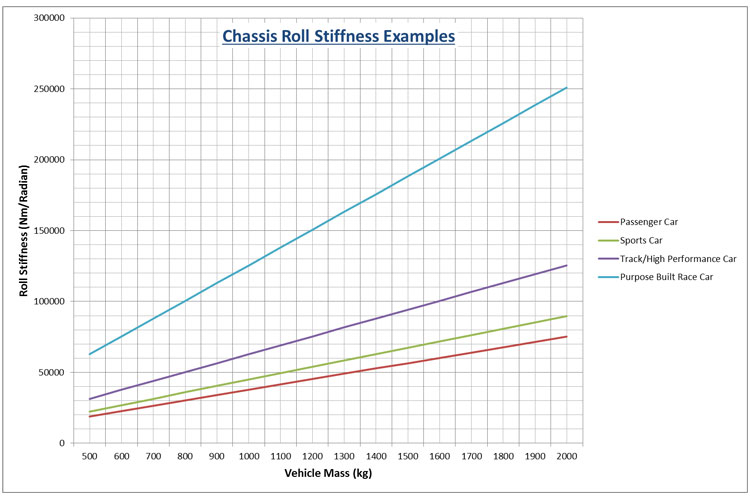Our vehicle mass is 1540 Kg  and is designed to be a purpose built race car which places us at 162700 Nm/radian on the graph. Next, the decision needs making how to split the overall chassis roll rate between the front and rear axles. Due to our example being rear wheel drive and front engined we are going to give the front wheels a higher roll rate than the rear to optimise rear wheel grip. For our example we are going to split the roll rate roughly 58/42 between our front and rear wheels giving the below values for our first iteration.

• Front roll rate (Kφf) = 94900 Nm/radian = 1656.3 Nm/degree
• Rear roll rate (Kφr) = 67800Nm/radian = 1183 Nm/degree

Due to us not yet knowing the ride rates we also don’t now the roll rates as they depend upon each other. Therefore we need to assume the roll rates to get the ball rolling for the calculations. We must also start the process at static values for roll centres due to them changing with ride height and roll angle changes ect.

Stage 2: The CalculationsThe first stage of the calculations is to calculate the longitudinal position of the centre of gravity position. For a more thorough calculation section on this, see “How to Calculate Centre of Gravity Position”. A more simple calculation can be seen below:And:Where:

• b = COG Position Distance From Rear Wheels (m)
• Wf = Front Axle Mass (kg)
• Wt= Total Vehicle Mass (kg)
• L = Wheel Base (m)
• a = COG Position Distance From Front Wheels (m)

Therefore:And:In this example the car is symmetrical so the COG position lies upon the centre line of the track width of the car. Now the lateral acceleration values can be calculated relative to the track angles and the earth. If you race on a banked or a flat circuit the values will differ here. Therefore we have carried out this next section twice. First we have used figures for a banked turn found in situations such as NASCAR. We have then carried out the same set up calculations for a car on a flat track such as Silverstone.  Just use the section that is applicable to your format of driving.

Banked Circuit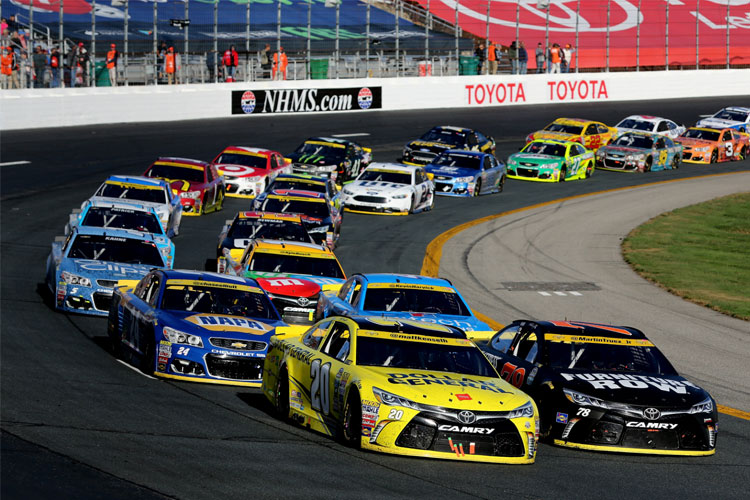For our example of a banked circuit we will use a banking angle (α) of 9.2 degrees for our calculations. This is the banking angle at the Indianapolis Motor Speedway used in NASCAR and INDY car racing.And:And:Where:

• Aα = Horizontal Lateral Acceleration (g)
• Ay = Lateral Acceleration (g)
• V = Cornering Velocity (m/s)
• R = Corner Radius (m)
• g = Gravity (m/s²)
• α = Banking Angle (degrees)
• W’ = Effective Weight of the Car Due to the Banking (kg)
• W = Total Static Mass of the Car (kg)

Therefore using our values from the first section we can run through these calculations:And:And: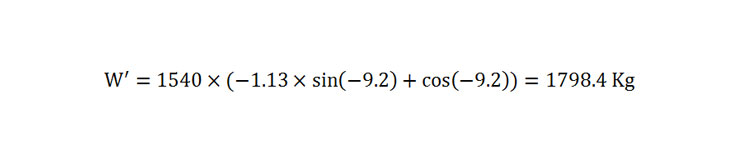With these figures we can now resume to the main calculation section. If you have used this banking section then skip the next part for calculating acceleration values on a flat circuit.

Flat CircuitWe can use the equations used for turning on a banked circuit to calculate the acceleration and load transfer rates on a flat circuit. As our banking angle (α) is equal to 0 degrees we can simply substitute out the following values in the equations to equal a whole number:

• Cosα =1
• Sinα = 0

With these two denotations we can generate the following equations:And:And:Where:

• Aα = Horizontal Lateral Acceleration (g)
• Ay = Lateral Acceleration (g)
• V = Cornering Velocity (m/s)
• R = Corner Radius (m)
• g = Gravity (m/s²)
• α = Banking Angle (0 degrees in this case)
• W’ = Effective Weight of the Car Due to the Banking (kg)
• W = Total Static Mass of the Car (kg)

Therefore using our values from the first section we can run through these calculations:And:And:As you can see the effective weight due to the banking is the same as the weight of the car due to there being no banking. However, we do now have our acceleration values for later use.

Resuming Main CalculationsWe can now calculate our effective front and rear axle weights due to cornering. Our example will continue using values for a flat circuit as that applies to the majority of vehicles in motorsport. However, if you are following this to set up an oval racing car then use the values from the banked circuit section.

The effective front and rear axle weights are:And:Where:

• W’F= Effective Front Axle Mass (kg)
• W’R = Effective Rear Axle Mass (kg)
• W’ = Total Effective Mass (kg)
• b = COG Position Distance From Rear Wheels (m)
• L = Wheel Base (m)
• a = COG Position Distance from Front Wheels (m)

Therefore using our values from above:And:Now we need to calculate our roll gradient using the equation:Where:

• Ѳ = Body Roll Angle (degrees)
• Ay = Lateral Acceleration (g)
• W = Total Vehicle Mass (kg)
• H = Centre of Gravity Distance to Roll Axis (m)
• KθF = Front Roll Rate (Nm/radian)
• KθR = Rear Roll Rate (Nm/radian)

Therefore:Now we calculate the front and rear lateral load transfer rates due to the lateral acceleration. The equations for this are:And:Where:

• Wf = Front Lateral Load Transfer (kg)
• Wr = Rear Lateral Load Transfer (kg)
• Ay = Lateral Acceleration (g)
• W = Total Vehicle Mass (kg)
• tf = Front Track Width (m)
• tr = Rear Track Width (m)
• H = Height of Centre of Gravity from Roll Axis (m)
• Kθf = Front Roll Rate (Radians/g)
• Kθr = Rear Roll Rate (Radians/g)
• b = COG Position Distance From Rear Wheels (m)
• L = Wheel Base (m)
• a = COG Position Distance from Front Wheels (m)
• Zrf = Front Roll Centre Height (m)
• Zrr = Rear Roll Centre Height (m)

Therefore we can use all of our figures from above to populate the equations and generate answers:And:Due to the magnitude of (Aαcosα) being greater than (sina) the outside wheel load of this car will increase and the inside wheel loads will reduce in mass; which is the normal way in which a car will load and unload wheels during cornering. Therefore, we can calculate the individual wheel loads for our car travelling around this corner.

• Front Outside Wheel = W(fo) = W1+Wf = 460+226.6 = 686.6 kg
• Front Inside Wheel = W(fi) = W2-Wf = 460-226.6 = 233.4 kg
• Rear Outside Wheel = W(ro) = W3 + Wr = 310+161.87 = 471.87 kg
• Rear Inside Wheel = W(ri) = W4 – Wr = 310-161.87 = 148.13 kg

If any of the above final values are negative or zero. This means that you are lifting that wheel off the ground. Now that we have the tyre loads for each wheel we can also calculate the change in tyre load from the static load for each wheel:

• Front Outside Wheel Change=ΔW(fo) = W(fo)-W1 =686.6 – 460= +226.6 kg
• Front Inside Wheel Change= ΔW(fi) =W(fi)-W2 =233.4 – 460= -226.6 kg
• Rear Outside Wheel Change = ΔW(ro) = W(ro)-W3 = 471.87 – 310= +161.87 kg
• Rear Inside Wheel Change = ΔW(ri) =W(ri)-W4 = 148.13 – 310 = -161.87 kg

You may notice that the above values are the same as calculated for effective wheel loads. However the numbers would be different if the banked corner example was used.

With our wheel loads generated, the next stage is to choose ride rates for our spring rates that are stiff enough so that our outside suspension does not bottom out or contact with bump stops. Therefore, if you calculated earlier that you have 90mm of bump travel available then it might be worth using 70mm to allow 20mm to account for extra unforeseen compression forces such as braking, acceleration and bumps in the circuit etc. It is very important to avoid bottoming out the wheel or the damper as this will cause a sudden rapid change in wheel loads as the spring rate will become ineffective if there is a mechanical locking in the system. In practicality bump stops and nonlinear rising rate springs can be used to overcome this but to keep the equations manageable we will assume that no bump stops are available and that fully linear springs are being used.If you have a car with adequate ground clearance then the main concern would be with the damper bottoming out under compression and contacting the bump stops. If the car is a ground effect car or used on a banked circuit at speed or both then the main concern could be the chassis bottoming out with the ground. Choose whichever scenario relates the most and decide upon how much bump travel is allowed. We will use the value of 70mm for this example.

Using our outside wheel loads from above and our allowed bump travel we can calculate the front and rear ride rates required:And:Where:

• Krf = Front Ride Rate (N/m)
• Krr = Rear Ride Rate (N/m)
• x1 = Front Bump Allowance (m)
• x2 = Rear Bump Allowance (m)

Therefore:And:Now we can calculate the ride frequencies continuing to assume side to side symmetry.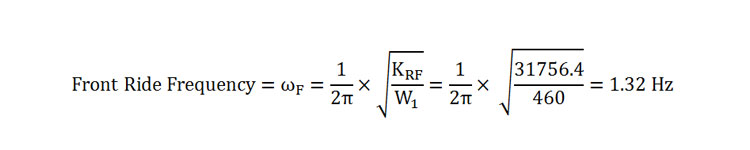And:To convert these ride frequencies to cycles per minute simply multiply by 60 to get:

• ωf = 1.32 Hz X 60 = 79.2cpm
• ωr = 1.36 Hz X 60 = 81.6 cpm

In this example we are looking at a rear wheel drive front engine race car. From experience and motorsport data we know that the front natural frequency is usually higher than that of the rear. In the above calculations this is not the case. Therefore we can say that front natural frequency must be higher than 81.6cpm and will therefore be increased to 86cpm for the first pass through the calculation. If you have a front wheel drive car then your rear natural frequency should be higher than your front. If it is not then you should do a similar process to the below but increasing your rear natural frequency not your front.

In order to increase the front natural frequency we can square the ratio of the two natural frequencies. Using the below equation:Using this ratio, the new front ride rate is: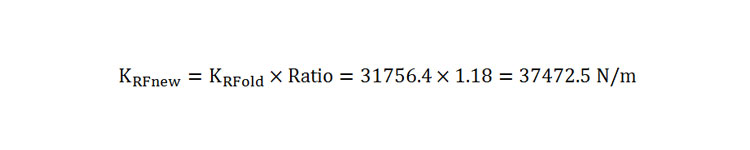To check that our new front ride rate has a higher natural frequency than the rear we can re-run the front natural frequency equation with our new front ride rate as below:The new answer for our front natural frequency is close enough to our approximation to continue with these values. With our first run through the calculations for ride rates complete we can now use these spring rate figures and compare them to the roll rates that were assumed at the beginning of the process to see how close we are. Assuming symmetry and that we want left and right ride rates to be the same the calculations for front and rear roll rates are:And:These values for roll are much lower than our assumed roll rates at the start of:

• Front roll rate = 94900 Nm/rad
• Rear roll rate = 67800 Nm/rad

Therefore the difference between these values is the required anti-roll bar spring rate for our front and rear axles. Therefore we can say that it is possible to install an anti-roll bar to our front and rear axles. The anti-roll bar spring rates required for now will be: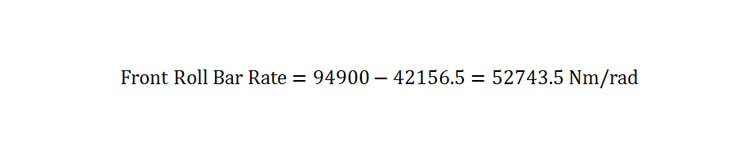AndIf you are unable to install a roll bar to your car at one end then you should continue the below few equations just using the coil spring roll rate for that end to see what effect it has upon wheel displacement. For interest reasons, if you did not have a car with side to side symmetry of ride rates such as an oval racing car then the below equation can be used to determine your front and rear roll rates:Now we can re-run some equations from earlier to check that our wheel ride displacements are as desired. First of all we must re-run our roll gradient equation from earlier with all 4 of our roll stiffness values:If you would like to convert your answer into degrees per g in order to compare your result with the earlier categorised values then the conversion formula below can be used.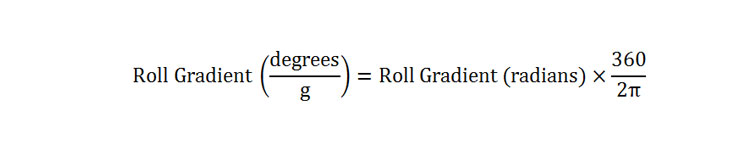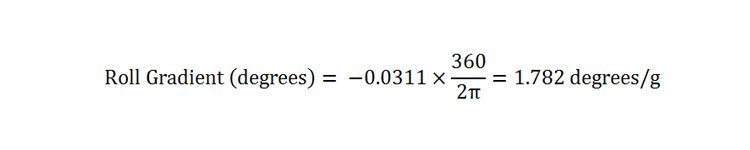Looking at the beginning of the process where we showed example roll gradients this falls at the stiff end of high performance road cars and track cars and just outside of the purpose built race car category. This is perfect for our example so we will continue with this value for now as the value will alter again slightly towards the end of the process.

And assuming roll centre heights are not altered, our front and rear weight transfer rates are:And:And our outside wheel loads are:

• Front Outside Wheel = W(fo) = W1+Wf = 460+226.6 = 686.6 kg
• Rear Outside Wheel = W(ro) = W3 + Wr = 310+161.87 = 471.87 kg

And our changes from static wheel loadings for our outside wheels are:

• Front Outside Wheel Change=ΔW(fo) = W(fo)-W1 =686.6 – 460= +226.6 kg
• Rear Outside Wheel Change = ΔW(ro) = W(ro)-W3 = 471.87 – 310= +161.87 kg

Therefore our wheel ride displacements are:And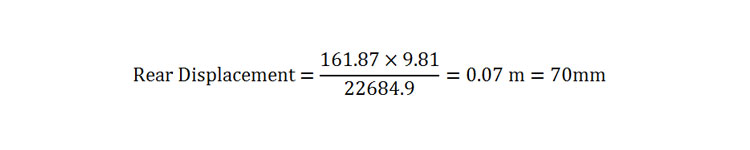Our front wheel displacement is close to what we initially wanted from our car but is a bit less. It is close enough to accept the front ride rate value for our example. The rear wheel displacement is exactly the same so we can use these figures for ride rates to move forward in our calculations. If you have carried numbers through such as where you were unable to install an anti-roll bar then your answers for wheel loads and wheel displacement may have altered.

This is a good point to check your roll gradient value and your ride frequency value with the figures at the beginning of the article to see if your values lie in the correct category of car. If it lies close to your category such as a fast track car that is close to a race car then leave your values. However if one or both of your answers puts you in the passenger car bracket when you are aiming to build a race car then you will need to alter your initial assumptions at the beginning and re-run the equations.

Furthermore, if you were not happy with your answers at this stage then you can return to the section of deciding upon wheel travel limits or if they are fixed you could look to altering items such as roll centre heights and track width which will alter earlier calculations and have a knock on effect up to this point. Only once you are happy with the above answers should you continue to the next section.

So far we have not accounted for tyres in the equations and much more, the next article will cover more features of geometry and tyres to produce our final answers for spring and roll bar rates.

Next Article:

Calculating Ideal Spring and Roll Bar Rates Part 2

Ref: Equations derived from: Milliken, W. and Milliken, D., 1995. Race Car Vehicle Dynamics. 1st ed. United States of America: SAE Publications Group.

### 2 thoughts on “Calculating Ideal Spring and Roll Bar Rates”

1.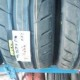Mohamed Anwar says:

Fantastic Article!!
but from the diagram in the beginning, the chassis roll stiffness closer to 192,700 right? vs 162,700 used in the calcuations or am i missing something?
Thanks again!

2.HARI KRISHNA says:

This data is very much useful for beginners.tq